﻿ 多功能共享单车机械结构设计与优化

多功能共享单车机械结构设计与优化Design and Optimization of Multi-Functional Shared Bicycle Mechanical Structure

Abstract: Due to the rapid development of the social economy, shared bicycle has become an indispensable tool for people to travel. Aiming at the problems of traditional shared bicycle structure, large parking space and limited storage space, this paper designs a multi-functional shared bicycle structure with foldable and deformable capabilities. Firstly, in order to ensure the function of the basket and the overall balance of the vehicle body when the multifunctional bicycle is deformed, a combined bicycle basket structure is designed by folding the bicycle handle, the seat and the aux-iliary device in the non-riding state. The bicycle frame under load is designed and checked for strength. Finally, ANSYS Workbench is used to simulate and optimize the bicycle structure, which makes the bicycle structure more concise and effective, greatly improves the performance and life, accelerates the use and promotion of folding shared bicycle, and meets the needs of larger society.

1. 引言

2. 多功能自行车机械结构设计

2.1. 多功能自行车的设计构想与思路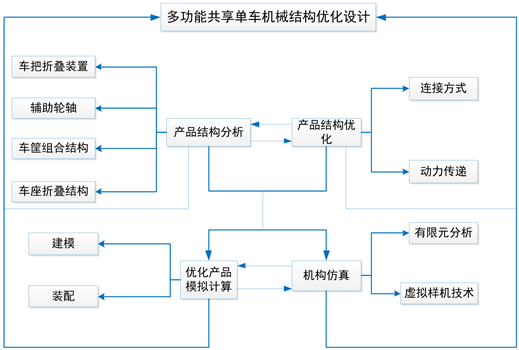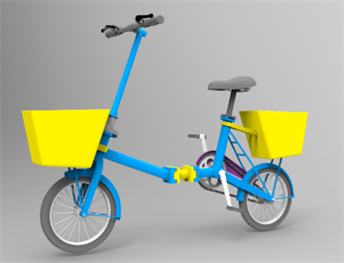Figure 2. Expanded state diagram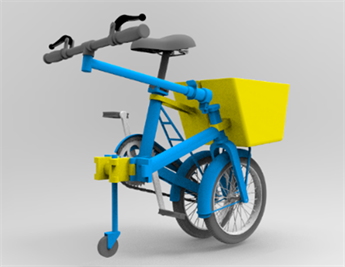Figure 3. Folded state diagram

2.2. 多功能自行车的结构和功能

2.2.1. 车把手折叠装置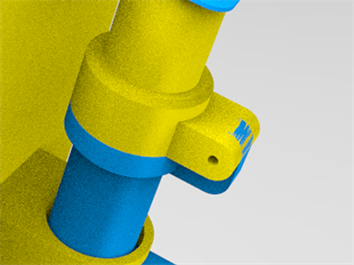Figure 4. Handlebar folding mechanism

2.2.2. 辅助轮轴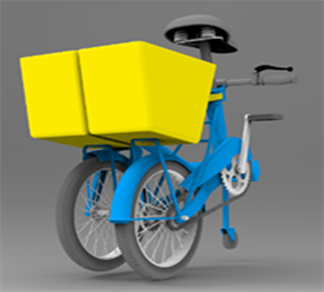(a)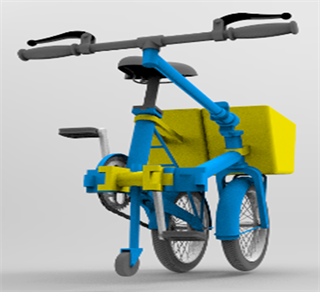(b)

Figure 5. Assembly drawing of auxiliary wheel contraction

2.2.3. 车筐组合结构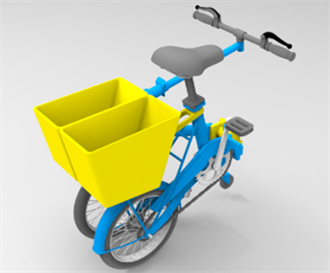Figure 6. Structure of combined basket

2.2.4. 车座折叠结构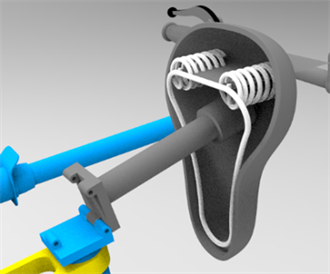2.3. 多功能自行车强度校核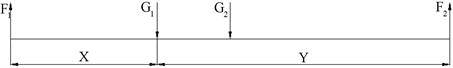Figure 8. Force diagram of bicycle

${F}_{1}={G}_{1}\cdot 720/1000+{G}_{2}\cdot 500/1000=543\text{\hspace{0.17em}}\text{N}$

${F}_{2}={G}_{1}\cdot 280/1000+{G}_{2}\cdot 500/1000=257\text{\hspace{0.17em}}\text{N}$

$F={F}_{1}\mathrm{cos}{45}^{\circ }=543×\mathrm{cos}{45}^{\circ }\approx 285\text{\hspace{0.17em}}\text{N}$

$M=FL=467×0.225=64.25\text{\hspace{0.17em}}\text{N}\cdot \text{m}$

$W=\frac{\pi {d}^{3}}{32}$

$\sigma =\frac{M}{W}=\frac{642.5}{{17}^{3}}\approx 130\text{\hspace{0.17em}}\text{MPa}$

${\sigma }_{-1}=320\text{\hspace{0.17em}}\text{MPa}$

3. 有限元仿真分析

3.1. 载荷工况分析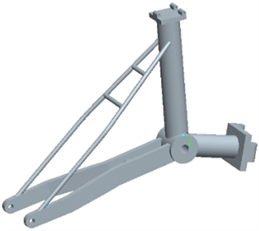Figure 9. Structure of rear support frame

3.2. 随机振动工况分析

3.2.1. 单位制确定及材料属性定义

3.2.2. 网格划分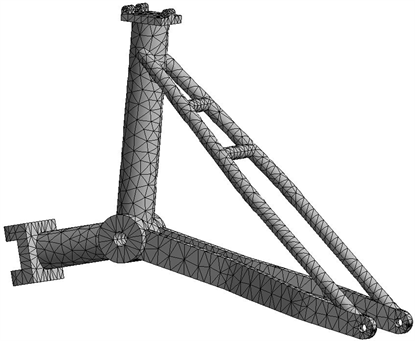Figure 10. Grid division of rear supporting frame

3.2.3. 载荷与边界条件设定

3.2.4. 计算结果及其评价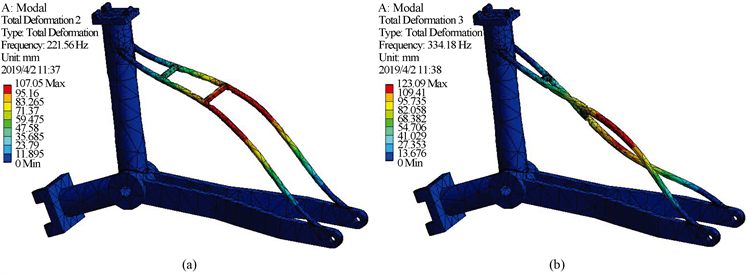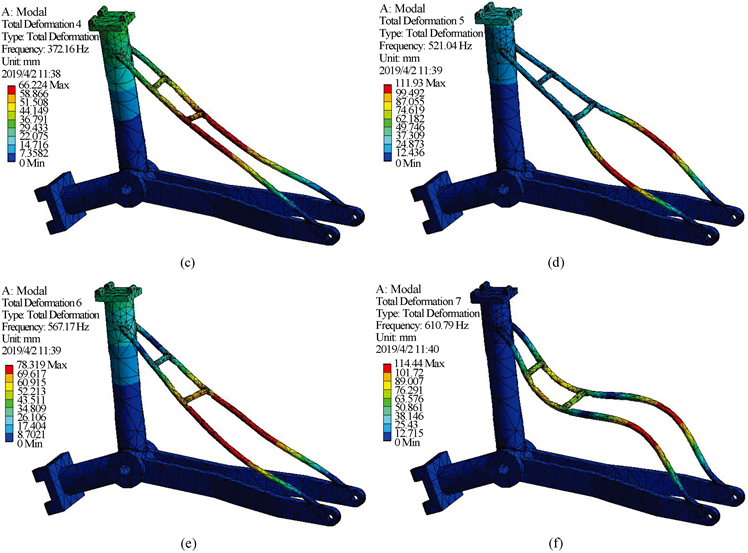Figure 11. Deformation cloud diagram of rear supporting frame

3.3. 优化设计方案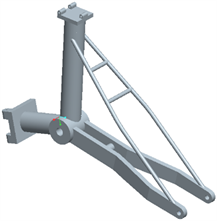Figure 12. Structure diagram before improvement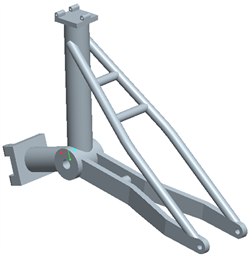Figure 13. Improved structure

3.4. 改进后随机振动工况分析

3.4.1. 单位制确定及材料属性定义

3.4.2. 网格的划分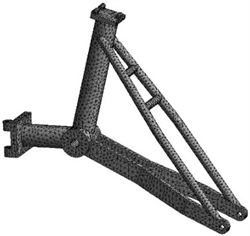Figure 14. Grid division of the improved supporting frame

3.4.3. 载荷与边界条件设定

3.4.4. 计算结果及其评价Figure 15. Deformation cloud diagram of the improved supporting frame

4. 结语

 魏静, 唐闯. “互联网+”模式下共享单车盈利模式探析[J]. 中国集体经济, 2019(9): 77-79.

 宋扬扬, 王胜本. “共享经济商业新范式——共享单车”的困境与治理[J]. 华北理工大学学报(社会科学版), 2019, 19(1): 24-28.

 陈晓航, 徐敏. 多功能购物自行车创新设计[J]. 科技创新与生产力, 2016(12): 96-97 + 100.

 陶松桥, 何伟. 多功能折叠自行车设计研究与探讨[J]. 轻工科技, 2012, 28(7): 68 + 117.

 蒋连琼, 沈志源. 折叠自行车的结构设计及三维建模[J]. 现代制造技术与装备, 2017(9): 99-100 + 102.

 李翔. 大型公共设施产生的自行车交通影响分析[J]. 交通与运输, 2004(6): 13.

 程宪春. 折叠自行车设计研究[J]. 包装工程, 2007, 28(8): 161-163.

 陈恳. 多功能提箱式折叠电动自行车[J]. 中国自行车, 2008(3): 31-34.

 罗红萍, 卢海燕. 新型多功能折叠自行车的设计计算研究[J]. 广西科技大学学报, 2014(2): 74-77.

 陈晓航, 徐敏. 多功能购物自行车创新设计[J]. 科技创新与生产力, 2016(12): 96-97 + 100.

Top